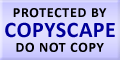Submit Assignment From Here
Name
:
Email
:
Phone
:
Country
:
Willing to pay US\$
:
Subject
:
Topic
:
Levels
:
:Hours
:

# Describing Data Visually Homework Help

A set of observation for a variable, a dot plot gets created which displays data for the variable, and then the frequency distribution divides the data into classes. These data classes are called bins. The Sturges’s Rule is as helpful component in making decision related to bins and their limits, but lastly bins and their limits are decisive upon the judgment of the user. The line chart depicts the value of more than one value of variable which are plotted against the time in the line chart. If there is variation in the data and the variation is due to magnitude then user needs to take the log scale. For the attributes the bar chart shows numerical data value. We can use bar chart in depicting time series too. We can see a relationship between two variables Y and Z with the help of a scattered diagram. The association between the two variables in question that is Y and Z. while we can see a numerical data value via many charts one of these is pie chart. A pie chart is a popular chart but it requires more research before it is used as compared to bar graphs or histograms. As there are common errors in the graphs the user should be aware of these types of errors.US